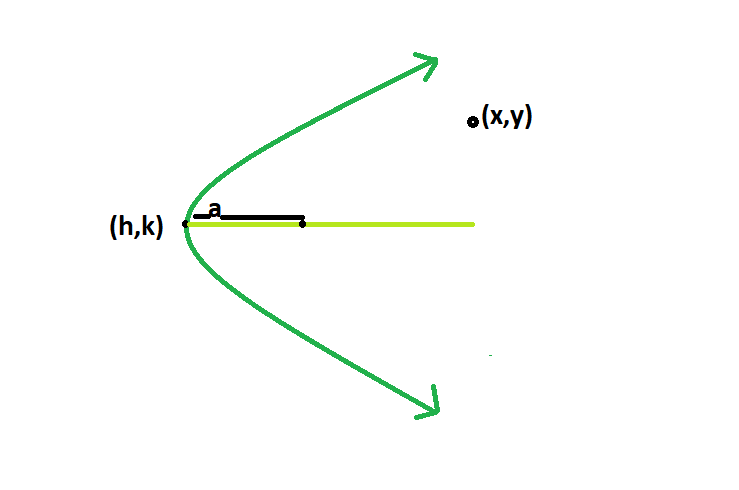# Check if a point is inside, outside or on the parabola

Given a parabola with vertex (h, k), and, the distance between focus and vertex. The task is to determine if the point (x, y) is inside, outside or on the parabola.

Examples:

```Input: h = 100, k = 500, x = 20, y = 10, a = 4
Output: Outside

Input: h = 0, k = 0, x = 2, y = 1, a = 4
Output: Inside
```

## Recommended: Please try your approach on {IDE} first, before moving on to the solution.Approach: It is very simple, we have to just solve the equation for point (x, y):

(y-k)^2 = 4a(x-h)
or, (y-k)^2 – 4a(x-h) = 0

After solving, if the result comes less than 0 then the point lies within, else if it comes exact 0 then the point lies on the parabola, and if the result is greater than 0 unsatisfied the point lies outside of the parabola.
Here we are taking a parabola whose axis of symmetry is y = k, although the approach is applicable for any parabola.

Below is the implementation of above approach:

## C++

 `// C++ Program to check if the point ` `// lies within the parabola or not ` `#include ` `using` `namespace` `std; ` ` `  `// Function to check the point ` `int` `checkpoint(``int` `h, ``int` `k, ``int` `x, ``int` `y, ``int` `a) ` `{ ` ` `  `    ``// checking the equation of ` `    ``// parabola with the given point ` `    ``int` `p = ``pow``((y - k), 2) - 4 * a * (x - h); ` ` `  `    ``return` `p; ` `} ` ` `  `// Driver code ` `int` `main() ` `{ ` `    ``int` `h = 0, k = 0, x = 2, y = 1, a = 4; ` ` `  `    ``if` `(checkpoint(h, k, x, y, a) > 0) ` `        ``cout << ``"Outside"` `<< endl; ` ` `  `    ``else` `if` `(checkpoint(h, k, x, y, a) == 0) ` `        ``cout << ``"On the parabola"` `<< endl; ` ` `  `    ``else` `        ``cout << ``"Inside"` `<< endl; ` ` `  `    ``return` `0; ` `} `

## Java

 `// Java Program to check if the point ` `// lies within the parabola or not ` ` `  `class` `solution ` `{ ` ` `  `// Function to check the point ` `static` `int` `checkpoint(``int` `h, ``int` `k, ``int` `x, ``int` `y, ``int` `a) ` `{ ` `    ``// checking the equation of ` `    ``// parabola with the given point ` `    ``int` `p =(``int``) Math.pow((y - k), ``2``) - ``4` `* a * (x - h); ` ` `  `    ``return` `p; ` `} ` ` `  `//driver code  ` `public` `static` `void` `main(String arr[]) ` `{ ` ` `  `    ``int` `h = ``0``, k = ``0``, x = ``2``, y = ``1``, a = ``4``; ` ` `  `    ``if` `(checkpoint(h, k, x, y, a) > ``0``) ` `    ``System.out.println(``"Outside"``); ` ` `  `    ``else` `if` `(checkpoint(h, k, x, y, a) == ``0``) ` `    ``System.out.println(``"On the parabola"``); ` ` `  `    ``else` `    ``System.out.println(``"Inside"``); ` ` `  `} ` `} `

## Python3

 `# Python3 Program to check if the point ` `# lies within the parabola or not ` ` `  `#  Function to check the point  ` `def` `checkpoint(h, k, x, y, a): ` ` `  `    ``# checking the equation of ` `    ``# parabola with the given point ` `    ``p ``=` `pow``((y ``-` `k), ``2``) ``-` `4` `*` `a ``*` `(x ``-` `h) ` ` `  `    ``return` `p ` ` `  `# Driver code ` `if` `__name__ ``=``=` `"__main__"` `:  ` `     `  `    ``h ``=` `0` `    ``k ``=` `0` `    ``x ``=` `2` `    ``y ``=` `1` `    ``a ``=` `4` ` `  `    ``if` `checkpoint(h, k, x, y, a) > ``0``: ` `        ``print` `(``"Outside\n"``) ` ` `  `    ``elif` `checkpoint(h, k, x, y, a) ``=``=` `0``: ` `        ``print` `(``"On the parabola\n"``) ` ` `  `    ``else``: ` `        ``print` `(``"Inside\n"``); ` `         `  `# This code is contributed by  ` `# Surendra_Gangwar `

## C#

 `// C# Program to check if the point  ` `// lies within the parabola or not  ` `using` `System; ` ` `  `class` `GFG ` `{ ` ` `  `// Function to check the point  ` `public` `static` `int` `checkpoint(``int` `h, ``int` `k, ` `                             ``int` `x, ``int` `y,  ` `                             ``int` `a) ` `{ ` `    ``// checking the equation of  ` `    ``// parabola with the given point  ` `    ``int` `p = (``int``) Math.Pow((y - k), 2) -  ` `                            ``4 * a * (x - h); ` ` `  `    ``return` `p; ` `} ` ` `  `// Driver code  ` `public` `static` `void` `Main(``string``[] arr) ` `{ ` `    ``int` `h = 0, k = 0,  ` `        ``x = 2, y = 1, a = 4; ` ` `  `    ``if` `(checkpoint(h, k, x, y, a) > 0) ` `    ``{ ` `        ``Console.WriteLine(``"Outside"``); ` `    ``} ` ` `  `    ``else` `if` `(checkpoint(h, k, x, y, a) == 0) ` `    ``{ ` `        ``Console.WriteLine(``"On the parabola"``); ` `    ``} ` ` `  `    ``else` `    ``{ ` `        ``Console.WriteLine(``"Inside"``); ` `    ``} ` `} ` `} ` ` `  `// This code is contributed  ` `// by Shrikant13 `

## PHP

 ` 0) ` `    ``echo` `"Outside"``; ` `else` `if` `(checkpoint(``\$h``, ``\$k``, ``\$x``,  ` `                    ``\$y``, ``\$a``) == 0) ` `    ``echo` `"On the parabola"``; ` `else` `    ``echo` `"Inside"``; ` ` `  `// This code is contributed  ` `// by inder_verma ` `?> `

Output:

```Inside
```

My Personal Notes arrow_drop_upCheck out this Author's contributed articles.

If you like GeeksforGeeks and would like to contribute, you can also write an article using contribute.geeksforgeeks.org or mail your article to contribute@geeksforgeeks.org. See your article appearing on the GeeksforGeeks main page and help other Geeks.

Please Improve this article if you find anything incorrect by clicking on the "Improve Article" button below.